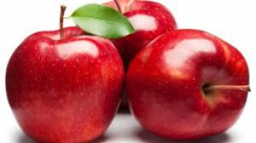The cost 2

The cost of 5 apples is $3.45, and 5 oranges are$1.23. If Rachel buys one apple and one orange, then how much must she pay?

x =  0.936 USD

Step-by-step explanation:Did you find an error or inaccuracy? Feel free to write us. Thank you!Tips to related online calculators
Need help to calculate sum, simplify or multiply fractions? Try our fraction calculator.
Do you want to perform natural numbers division - find the quotient and remainder?